# NCERT Solutions for Class 9 Maths Chapter 12 - Heron's Formula

Share

NCERT Solutions For Class 9 Maths Chapter 12 Heron’s formula provided by Goprep act as a tailor-made resource for the exam preparation. So, students who are facing difficulty in understanding the various concepts explained in Chapter 12 of the textbook can simply refer to these Solutions to aid their exam preparation.

Further, these Solutions have been prepared by expert teachers according to the prevailing syllabus of the CBSE which all the more enhances their value. The Chapter 12 of the Maths textbook of Class 9 discusses the important concept of Heron’s Formula, its Introduction, Area Of A Triangle – By Heron’s Formula, Application Of Heron’s Formula In Finding Areas Of Quadrilaterals. So, students who want to get hold of the important topics quickly without much hassle can refer to our NCERT Solutions for Chapter 12 to perform better in the exam.

## NCERT Solutions for Class 9 Maths Chapter 12 - Heron's Formula

Exercise 12.1
• Exercise 12.1
• Exercise 12.2
Class 9th|NCERT - MathematicsChapter 12 - Heron's Formula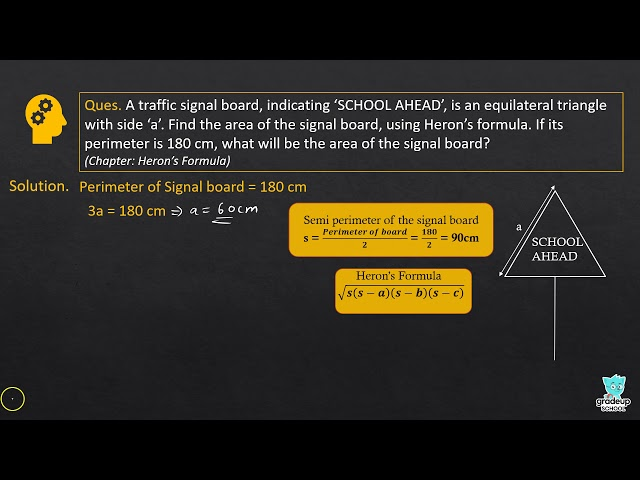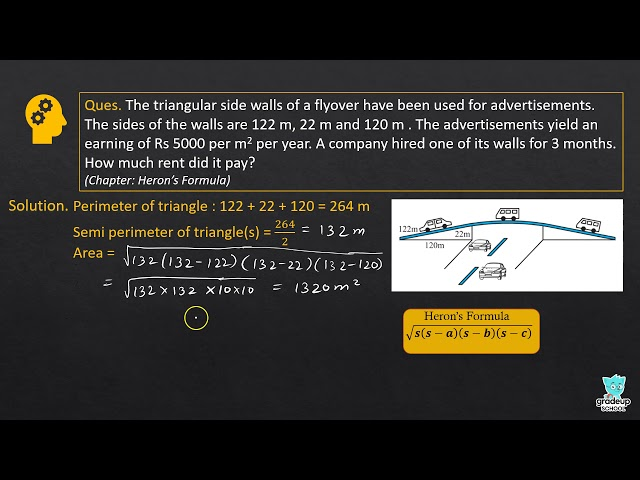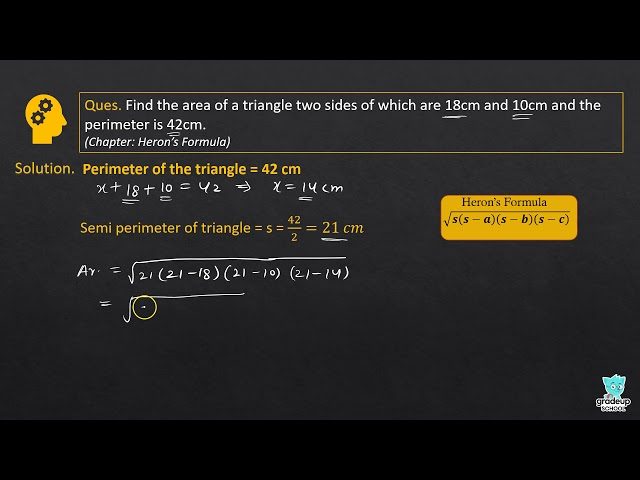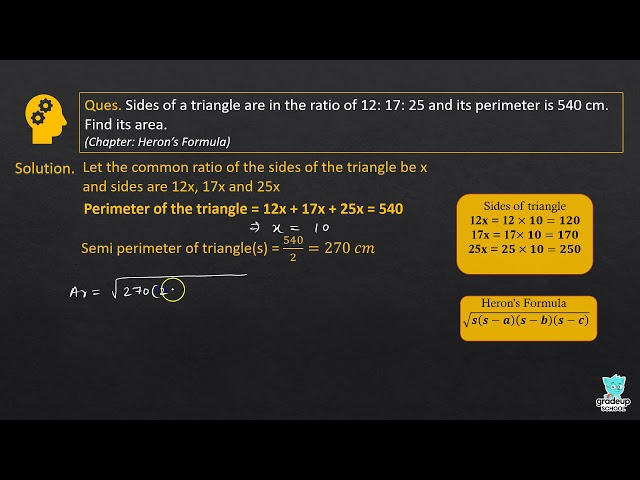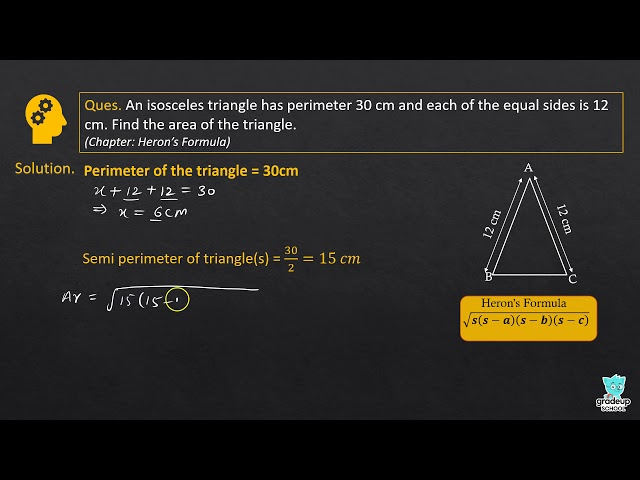NCERT - MathematicsClass 9th , NCERT
 Chapter 1 - Number System Chapter 2 - Polynomials Chapter 3 - Coordinate Geometry Chapter 4 - Linear Equations in two Variables Chapter 5 - Introduction to Euclid's Geometry Chapter 6 - Lines and Angles Chapter 7 - Triangles Chapter 8 - Quadrilaterals Chapter 9 - Areas of Parallelograms and Triangles Chapter 10 - Circles Chapter 11 - Constructions Chapter 12 - Heron's Formula Chapter 13 - Surface Areas and Volumes Chapter 14 - Statistics Chapter 15 - Probability